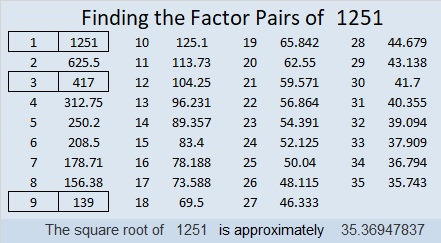# 1251 and Level 1

Other than 1, what is the common factor of all the clues in this puzzle? Use that answer to fill in all the cells in the first column and the top row with the numbers from 1 to 12. then you will have the start of a different kind of multiplication table.Print the puzzles or type the solution in this excel file: 12 factors 1251-1258

Now I’ll share some facts about the number 1251:

• 1251 is a composite number.
• Prime factorization: 1251 = 3 × 3 × 139, which can be written 1251 = 3² × 139
• The exponents in the prime factorization are 2 and 1. Adding one to each and multiplying we get (2 + 1)(1 + 1) = 3 × 2  = 6. Therefore 1251 has exactly 6 factors.
• Factors of 1251: 1, 3, 9, 139, 417, 1251
• Factor pairs: 1251 = 1 × 1251, 3 × 417, or 9 × 139
• Taking the factor pair with the largest square number factor, we get √1251 = (√9)(√139) = 3√139 ≈ 35.369481251 is also the sum of five consecutive prime numbers:
239 + 241 + 251 + 257 + 263 = 1251